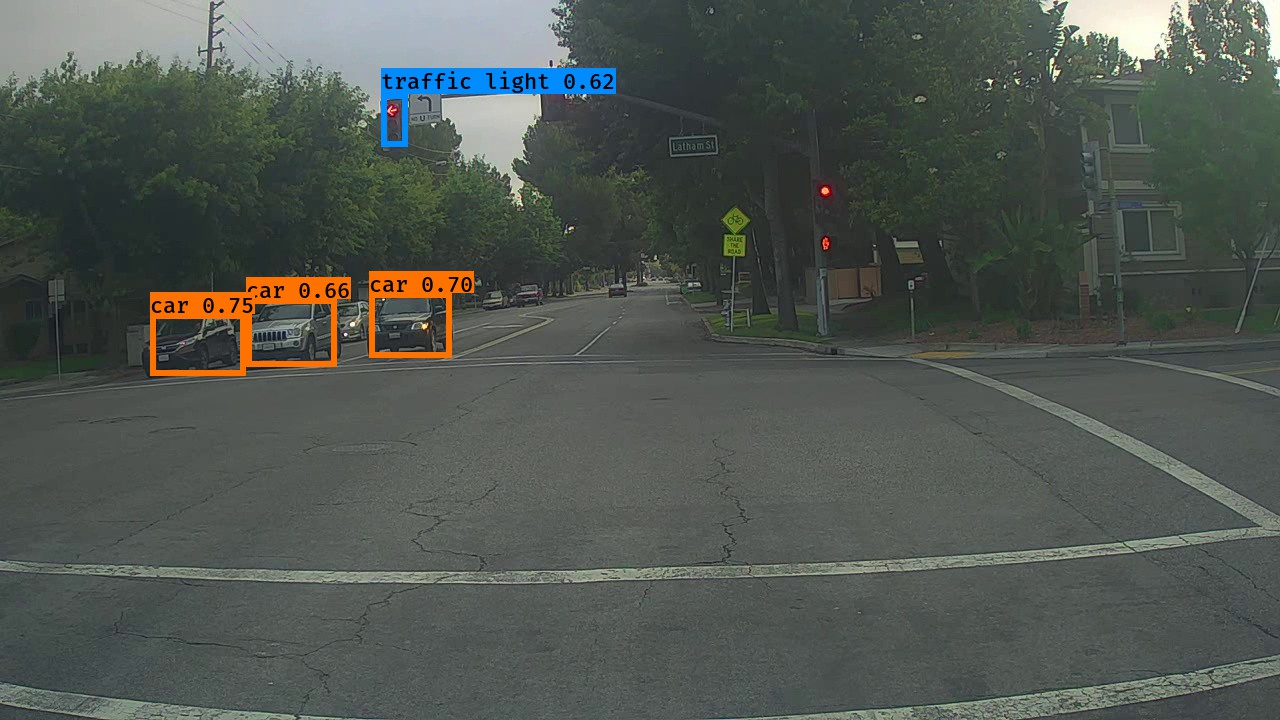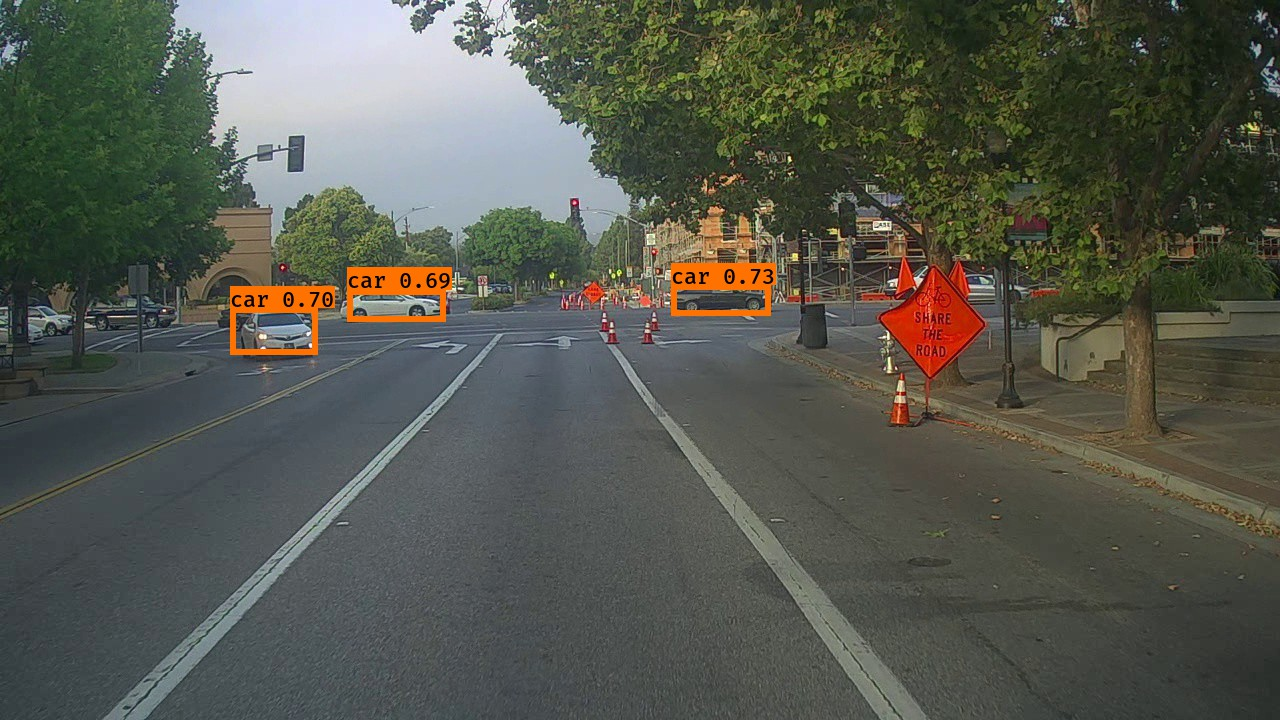# Autonomous driving - Car detection

Welcome to your week 3 programming assignment. You will learn about object detection using the very powerful YOLO model. Many of the ideas in this notebook are described in the two YOLO papers: Redmon et al., 2016 (https://arxiv.org/abs/1506.02640) and Redmon and Farhadi, 2016 (https://arxiv.org/abs/1612.08242).

You will learn to:

• Use object detection on a car detection dataset
• Deal with bounding boxes

Run the following cell to load the packages and dependencies that are going to be useful for your journey!

import argparse
import os
import keras
import matplotlib.pyplot as plt
from matplotlib.pyplot import imshow
import scipy.io
import scipy.misc
import numpy as np
import pandas as pd
import PIL
import tensorflow as tf
from IPython.display import SVG
from keras.utils.vis_utils import model_to_dot
from keras import backend as K
from keras.layers import Input, Lambda, Conv2D
from keras.models import load_model, Model
from yolo_utils import read_classes, read_anchors, generate_colors, preprocess_image, draw_boxes, scale_boxes
from yad2k.models.keras_yolo import yolo_head, yolo_boxes_to_corners, preprocess_true_boxes, yolo_loss, yolo_body

%matplotlib inline


Important Note: As you can see, we import Keras’s backend as K. This means that to use a Keras function in this notebook, you will need to write: K.function(...).

## 1 - Problem Statement

You are working on a self-driving car. As a critical component of this project, you’d like to first build a car detection system. To collect data, you’ve mounted a camera to the hood (meaning the front) of the car, which takes pictures of the road ahead every few seconds while you drive around.

You’ve gathered all these images into a folder and have labelled them by drawing bounding boxes around every car you found. Here’s an example of what your bounding boxes look like.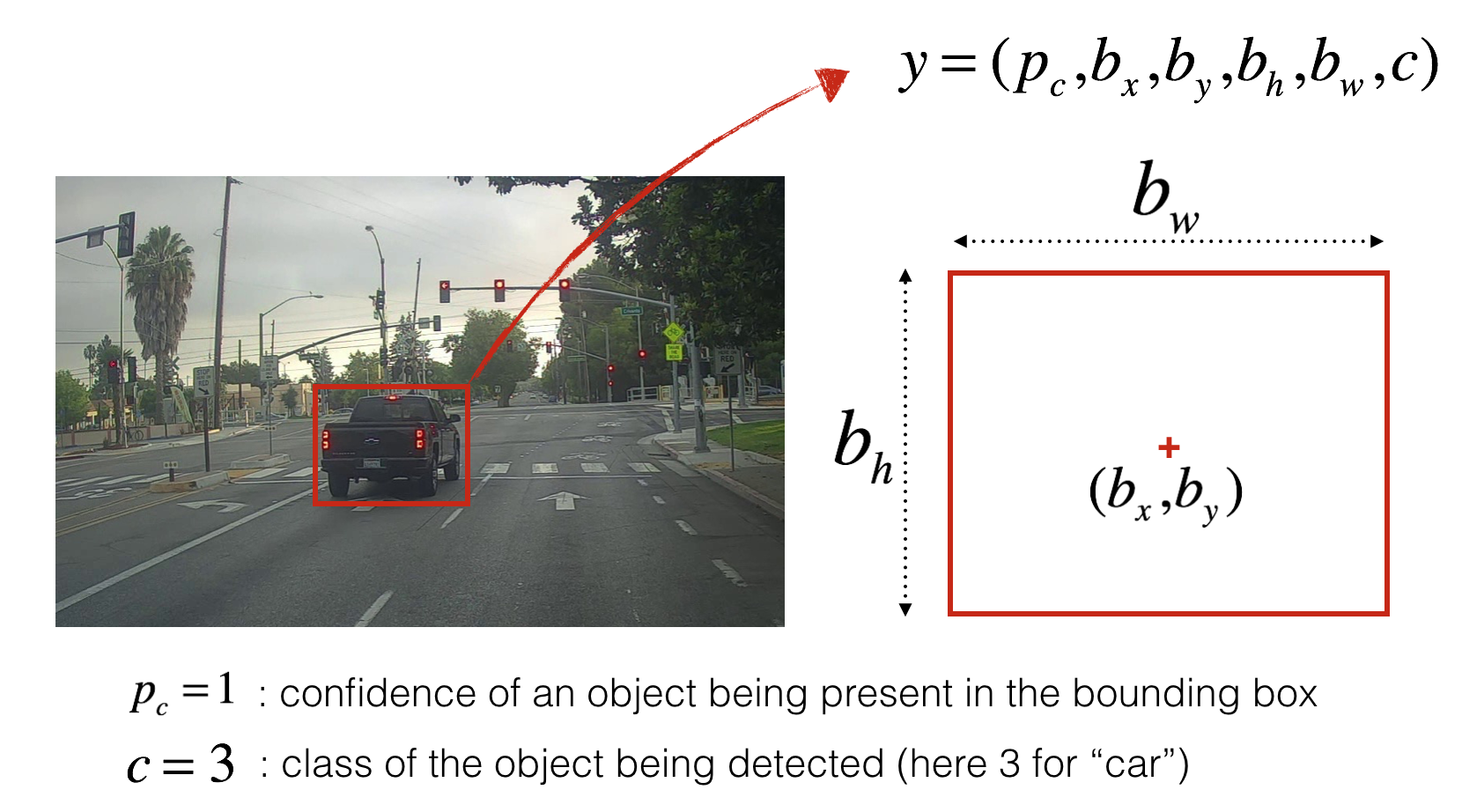If you have 80 classes that you want YOLO to recognize, you can represent the class label $c$ either as an integer from 1 to 80, or as an 80-dimensional vector (with 80 numbers) one component of which is 1 and the rest of which are 0. The video lectures had used the latter representation; in this notebook, we will use both representations, depending on which is more convenient for a particular step.

In this exercise, you will learn how YOLO works, then apply it to car detection. Because the YOLO model is very computationally expensive to train, we will load pre-trained weights for you to use.

## 2 - YOLO

YOLO (“you only look once”) is a popular algoritm because it achieves high accuracy while also being able to run in real-time. This algorithm “only looks once” at the image in the sense that it requires only one forward propagation pass through the network to make predictions. After non-max suppression, it then outputs recognized objects together with the bounding boxes.

### 2.1 - Model details

First things to know:

• The input is a batch of images of shape (m, 608, 608, 3)
• The output is a list of bounding boxes along with the recognized classes. Each bounding box is represented by 6 numbers $(p_c, b_x, b_y, b_h, b_w, c)$ as explained above. If you expand $c$ into an 80-dimensional vector, each bounding box is then represented by 85 numbers.

We will use 5 anchor boxes. So you can think of the YOLO architecture as the following: IMAGE (m, 608, 608, 3) -> DEEP CNN -> ENCODING (m, 19, 19, 5, 85).

Lets look in greater detail at what this encoding represents.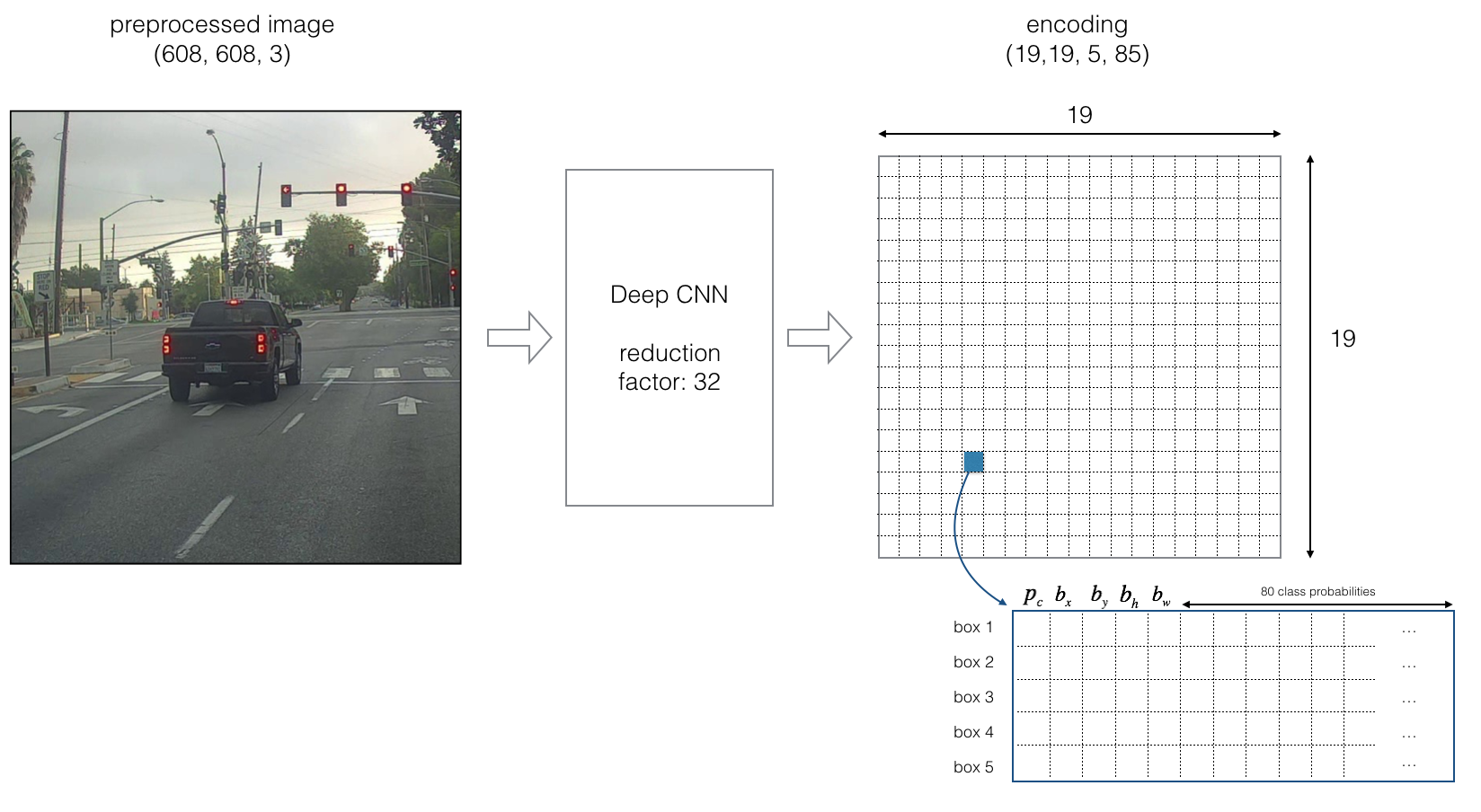If the center/midpoint of an object falls into a grid cell, that grid cell is responsible for detecting that object.

Since we are using 5 anchor boxes, each of the 19 x19 cells thus encodes information about 5 boxes. Anchor boxes are defined only by their width and height.

For simplicity, we will flatten the last two last dimensions of the shape (19, 19, 5, 85) encoding. So the output of the Deep CNN is (19, 19, 425).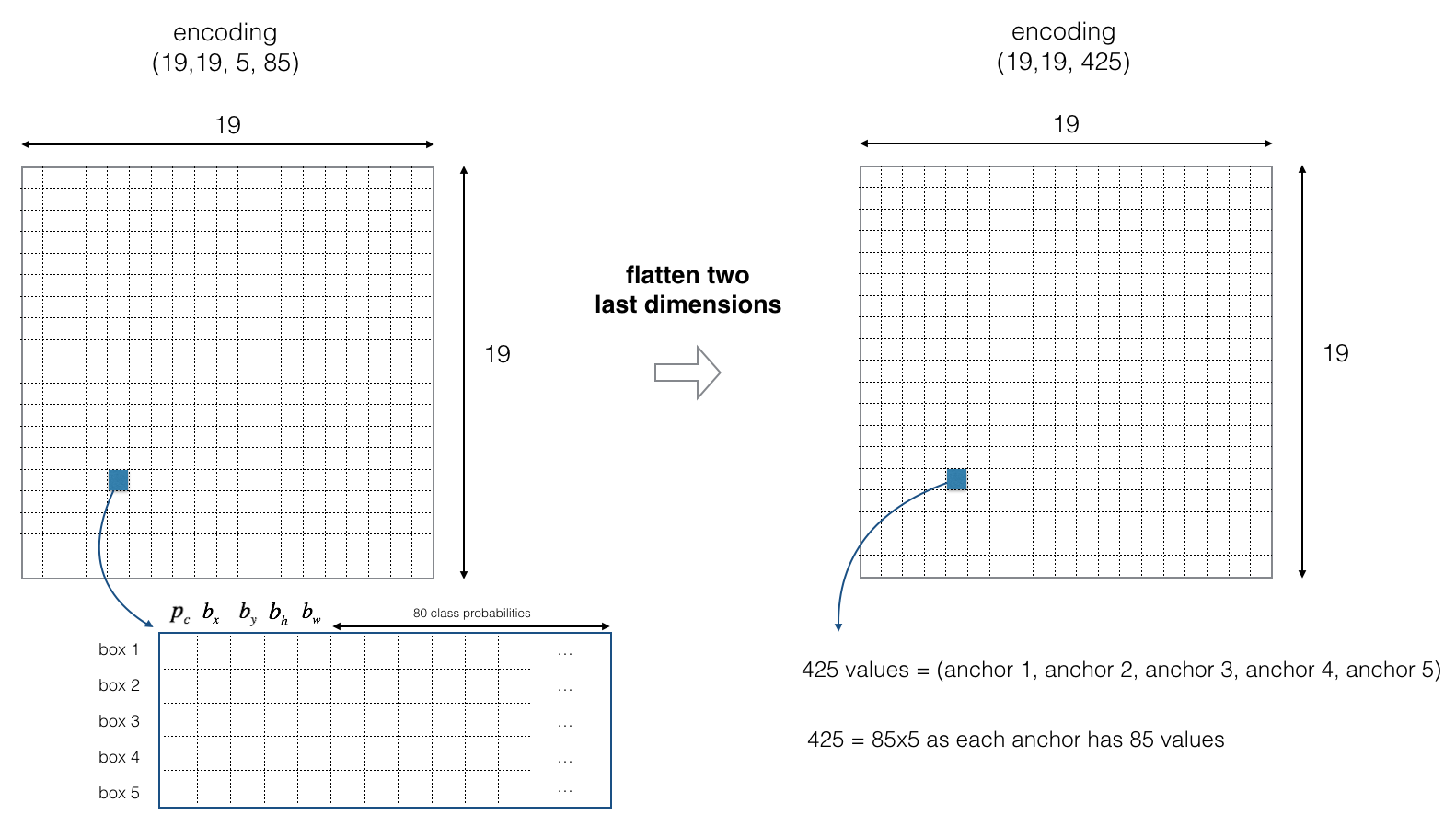Figure 3 : Flattening the last two last dimensions

Now, for each box (of each cell) we will compute the following elementwise product and extract a probability that the box contains a certain class.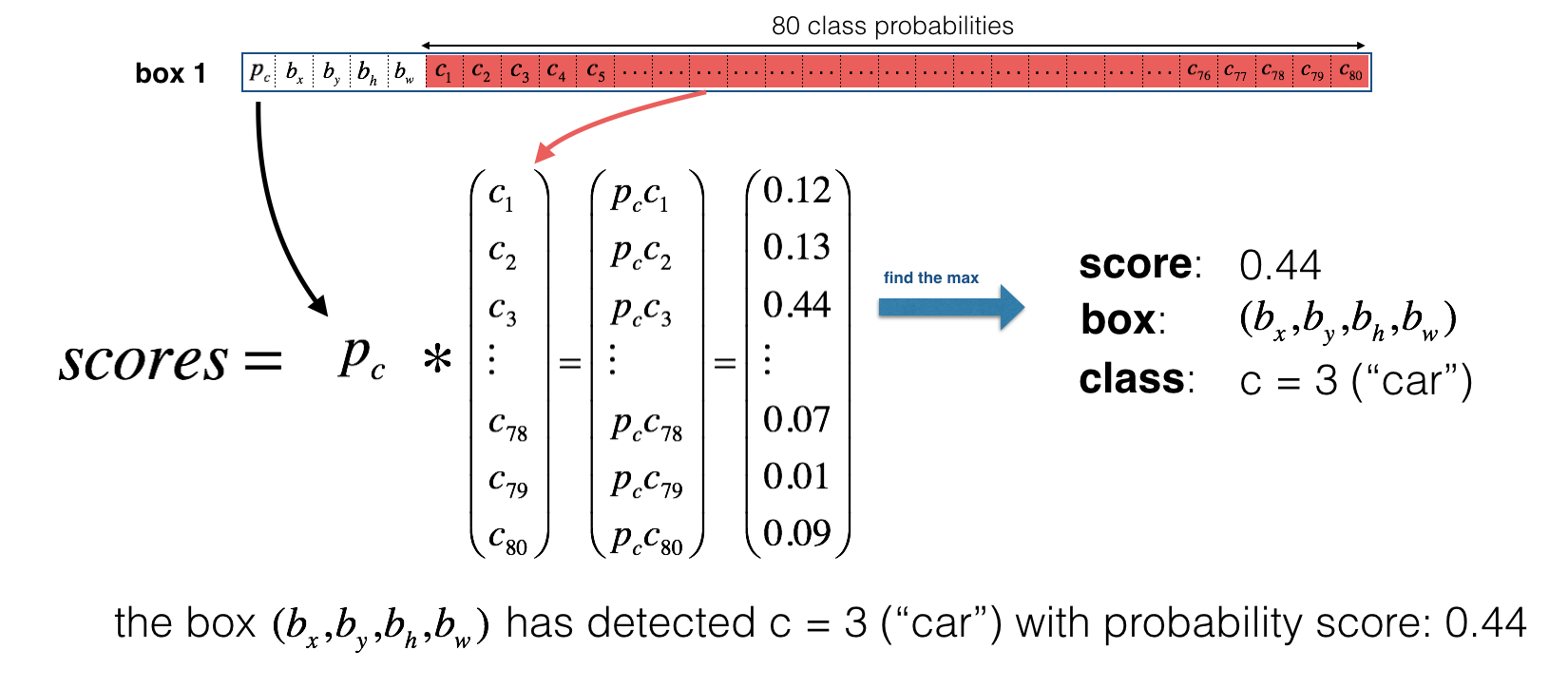Here’s one way to visualize what YOLO is predicting on an image:

• For each of the 19x19 grid cells, find the maximum of the probability scores (taking a max across both the 5 anchor boxes and across different classes).
• Color that grid cell according to what object that grid cell considers the most likely.

Doing this results in this picture: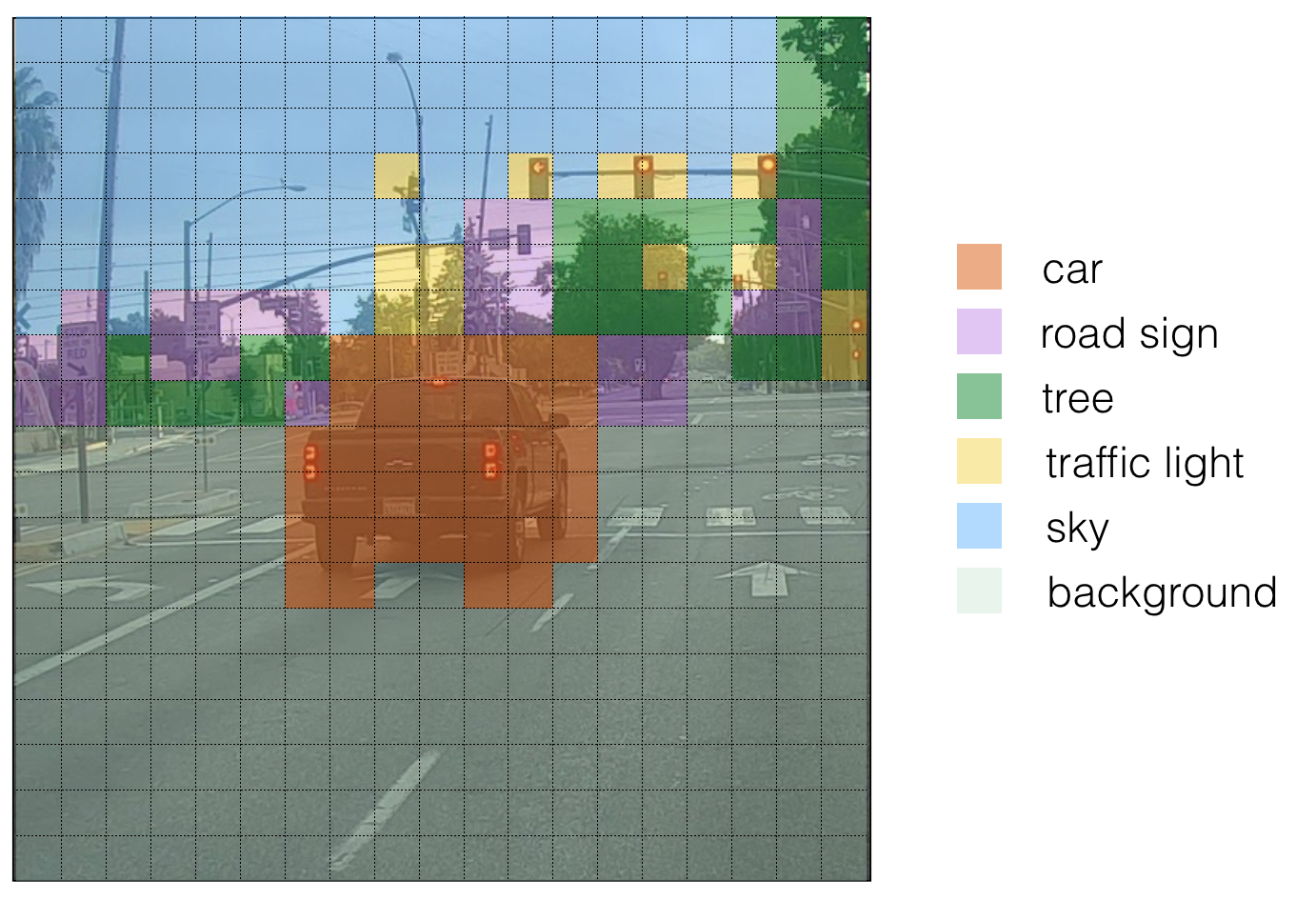Figure 5 : Each of the 19x19 grid cells colored according to which class has the largest predicted probability in that cell.

Note that this visualization isn’t a core part of the YOLO algorithm itself for making predictions; it’s just a nice way of visualizing an intermediate result of the algorithm.
Another way to visualize YOLO’s output is to plot the bounding boxes that it outputs. Doing that results in a visualization like this: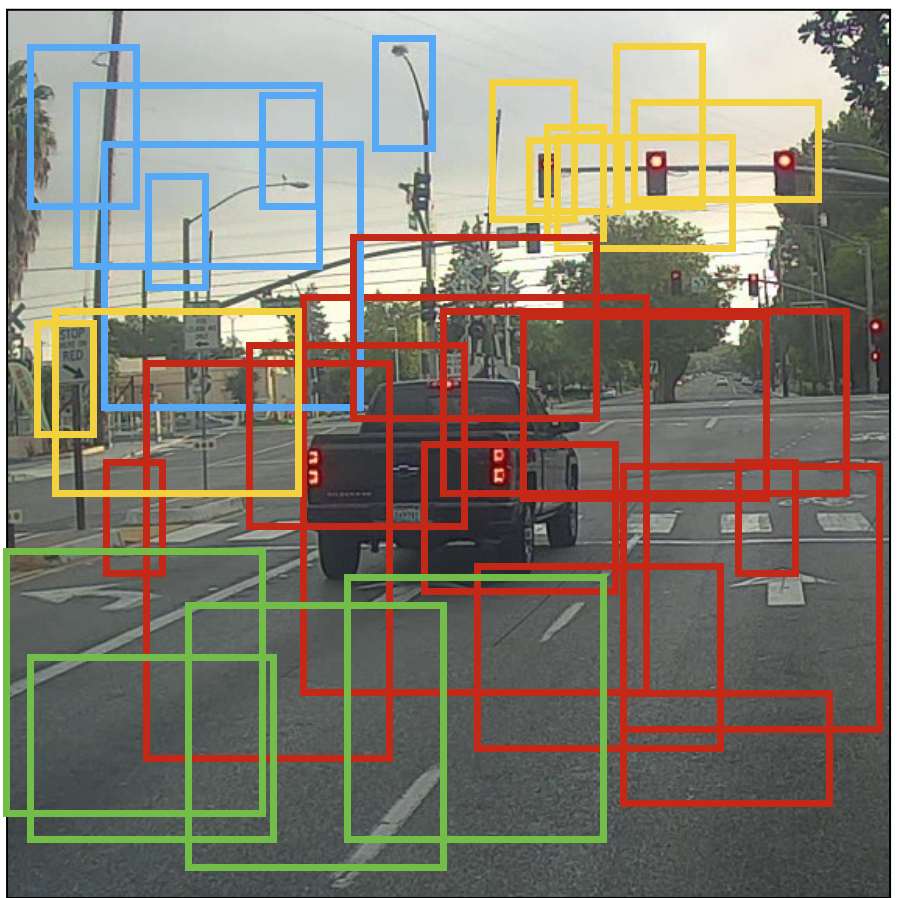**Figure 6** : Each cell gives you 5 boxes. In total, the model predicts: 19x19x5 = 1805 boxes just by looking once at the image (one forward pass through the network)! Different colors denote different classes.

In the figure above, we plotted only boxes that the model had assigned a high probability to, but this is still too many boxes. You’d like to filter the algorithm’s output down to a much smaller number of detected objects. To do so, you’ll use non-max suppression. Specifically, you’ll carry out these steps:

• Get rid of boxes with a low score (meaning, the box is not very confident about detecting a class)
• Select only one box when several boxes overlap with each other and detect the same object.

### 2.2 - Filtering with a threshold on class scores

You are going to apply a first filter by thresholding. You would like to get rid of any box for which the class “score” is less than a chosen threshold.

The model gives you a total of 19x19x5x85 numbers, with each box described by 85 numbers. It’ll be convenient to rearrange the (19,19,5,85) (or (19,19,425)) dimensional tensor into the following variables:

• box_confidence: tensor of shape $(19 \times 19, 5, 1)$ containing $p_c$ (confidence probability that there’s some object) for each of the 5 boxes predicted in each of the 19x19 cells.
• boxes: tensor of shape $(19 \times 19, 5, 4)$ containing $(b_x, b_y, b_h, b_w)$ for each of the 5 boxes per cell.
• box_class_probs: tensor of shape $(19 \times 19, 5, 80)$ containing the detection probabilities $(c_1, c_2, ... c_{80})$ for each of the 80 classes for each of the 5 boxes per cell.

Exercise: Implement yolo_filter_boxes().

1. Compute box scores by doing the elementwise product as described in Figure 4. The following code may help you choose the right operator:
a = np.random.randn(19*19, 5, 1)
b = np.random.randn(19*19, 5, 80)
c = a * b # shape of c will be (19*19, 5, 80)

1. For each box, find:
• the index of the class with the maximum box score (Hint) (Be careful with what axis you choose; consider using axis=-1)
• the corresponding box score (Hint) (Be careful with what axis you choose; consider using axis=-1)
2. Create a mask by using a threshold. As a reminder: ([0.9, 0.3, 0.4, 0.5, 0.1] < 0.4) returns: [False, True, False, False, True]. The mask should be True for the boxes you want to keep.
3. Use TensorFlow to apply the mask to box_class_scores, boxes and box_classes to filter out the boxes we don’t want. You should be left with just the subset of boxes you want to keep. (Hint)

Reminder: to call a Keras function, you should use K.function(...).

def yolo_filter_boxes(box_confidence, boxes, box_class_probs, threshold = .6):
"""Filters YOLO boxes by thresholding on object and class confidence.

Arguments:
box_confidence -- tensor of shape (19, 19, 5, 1)
boxes -- tensor of shape (19, 19, 5, 4)
box_class_probs -- tensor of shape (19, 19, 5, 80)
threshold -- real value, if [ highest class probability score < threshold], then get rid of the corresponding box

Returns:
scores -- tensor of shape (None,), containing the class probability score for selected boxes
boxes -- tensor of shape (None, 4), containing (b_x, b_y, b_h, b_w) coordinates of selected boxes
classes -- tensor of shape (None,), containing the index of the class detected by the selected boxes

Note: "None" is here because you don't know the exact number of selected boxes, as it depends on the threshold.
For example, the actual output size of scores would be (10,) if there are 10 boxes.
"""

# Step 1: Compute box scores
### START CODE HERE ### (≈ 1 line)
box_scores = np.multiply(box_confidence,box_class_probs)
### END CODE HERE ###

# Step 2: Find the box_classes thanks to the max box_scores, keep track of the corresponding score
### START CODE HERE ### (≈ 2 lines)
box_classes = K.argmax(box_scores,axis=-1)  # shape (19,19,5)
box_class_scores = K.max(box_scores,axis=-1)  # shape (19,19,5)
### END CODE HERE ###

# Step 3: Create a filtering mask based on "box_class_scores" by using "threshold". The mask should have the
# same dimension as box_class_scores, and be True for the boxes you want to keep (with probability >= threshold)
### START CODE HERE ### (≈ 1 line)
filtering_mask = box_class_scores >= threshold  #判断分数，将大于阈值的置1，小于值的置 0
### END CODE HERE ###

# Step 4: Apply the mask to scores, boxes and classes
### START CODE HERE ### (≈ 3 lines)
scores = tf.boolean_mask(box_class_scores,filtering_mask)
boxes = tf.boolean_mask(boxes,filtering_mask)
classes = tf.boolean_mask(box_classes,filtering_mask)
### END CODE HERE ###

return scores, boxes, classes


### 2.3 - Non-max suppression

Even after filtering by thresholding over the classes scores, you still end up a lot of overlapping boxes. A second filter for selecting the right boxes is called non-maximum suppression (NMS).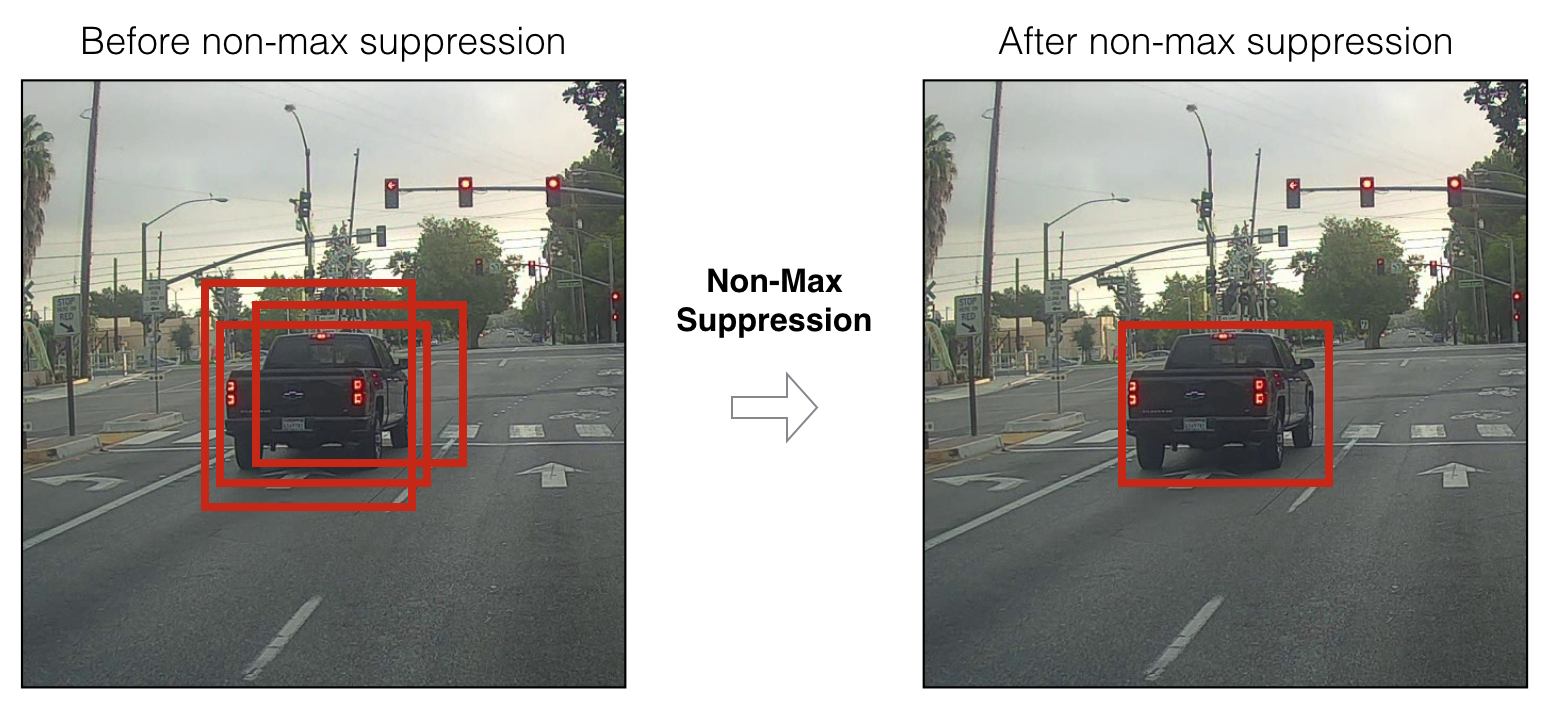**Figure 7** : In this example, the model has predicted 3 cars, but it's actually 3 predictions of the same car. Running non-max suppression (NMS) will select only the most accurate (highest probabiliy) one of the 3 boxes.

Non-max suppression uses the very important function called “Intersection over Union”, or IoU.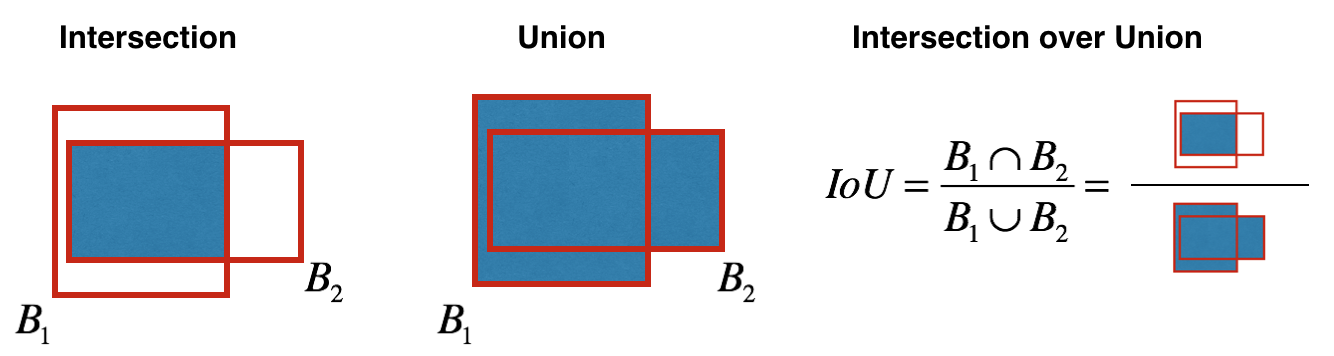**Figure 8** : Definition of "Intersection over Union".

Exercise: Implement iou(). Some hints:

• In this exercise only, we define a box using its two corners (upper left and lower right): (x1, y1, x2, y2) rather than the midpoint and height/width.
• To calculate the area of a rectangle you need to multiply its height (y2 - y1) by its width (x2 - x1)
• You’ll also need to find the coordinates (xi1, yi1, xi2, yi2) of the intersection of two boxes. Remember that:
• xi1 = maximum of the x1 coordinates of the two boxes
• yi1 = maximum of the y1 coordinates of the two boxes
• xi2 = minimum of the x2 coordinates of the two boxes
• yi2 = minimum of the y2 coordinates of the two boxes

In this code, we use the convention that (0,0) is the top-left corner of an image, (1,0) is the upper-right corner, and (1,1) the lower-right corner.

def iou(box1, box2):
"""Implement the intersection over union (IoU) between box1 and box2

Arguments:
box1 -- first box, list object with coordinates (x1, y1, x2, y2)
box2 -- second box, list object with coordinates (x1, y1, x2, y2)
"""

# Calculate the (y1, x1, y2, x2) coordinates of the intersection of box1 and box2. Calculate its Area.
### START CODE HERE ### (≈ 5 lines)
xi1 = np.maximum(box1,box2)
yi1 = np.maximum(box1,box2)
xi2 = np.minimum(box1,box2)
yi2 = np.minimum(box1,box2)
inter_area = (xi2-xi1)*(yi2-yi1)
### END CODE HERE ###

# Calculate the Union area by using Formula: Union(A,B) = A + B - Inter(A,B)
### START CODE HERE ### (≈ 3 lines)
box1_area = (box1-box1)*(box1-box1)
box2_area = (box2-box2)*(box2-box2)
union_area =(box1_area+box2_area)-inter_area
### END CODE HERE ###

# compute the IoU
### START CODE HERE ### (≈ 1 line)
iou = inter_area/union_area
### END CODE HERE ###

return iou


ou are now ready to implement non-max suppression. The key steps are:

1. Select the box that has the highest score.
2. Compute its overlap with all other boxes, and remove boxes that overlap it more than iou_threshold.
3. Go back to step 1 and iterate until there’s no more boxes with a lower score than the current selected box.

This will remove all boxes that have a large overlap with the selected boxes. Only the “best” boxes remain.

Exercise: Implement yolo_non_max_suppression() using TensorFlow. TensorFlow has two built-in functions that are used to implement non-max suppression (so you don’t actually need to use your iou() implementation):

def yolo_non_max_suppression(scores, boxes, classes, max_boxes = 10, iou_threshold = 0.5):
"""
Applies Non-max suppression (NMS) to set of boxes

Arguments:
scores -- tensor of shape (None,), output of yolo_filter_boxes()
boxes -- tensor of shape (None, 4), output of yolo_filter_boxes() that have been scaled to the image size (see later)
classes -- tensor of shape (None,), output of yolo_filter_boxes()
max_boxes -- integer, maximum number of predicted boxes you'd like,想要输出的box的最大数量
iou_threshold -- real value, "intersection over union" threshold used for NMS filtering

Returns:
scores -- tensor of shape (, None), predicted score for each box  #这里的维度也改变了
boxes -- tensor of shape (4, None), predicted box coordinates
classes -- tensor of shape (, None), predicted class for each box

Note: The "None" dimension of the output tensors has obviously to be less than max_boxes. Note also that this
function will transpose the shapes of scores, boxes, classes. This is made for convenience.
"""

max_boxes_tensor = K.variable(max_boxes, dtype='int32')     # tensor to be used in tf.image.non_max_suppression()
K.get_session().run(tf.variables_initializer([max_boxes_tensor])) # initialize variable max_boxes_tensor

# Use tf.image.non_max_suppression() to get the list of indices corresponding to boxes you keep
### START CODE HERE ### (≈ 1 line)
nms_indices = tf.image.non_max_suppression(boxes,scores,max_boxes,iou_threshold)  #返回的是索引，boxes/scores
### END CODE HERE ###
# Use K.gather() to select only nms_indices from scores, boxes and classes
### START CODE HERE ### (≈ 3 lines)
scores = K.gather(scores,nms_indices)   #保留nms_indices对应的索引
boxes = K.gather(boxes,nms_indices)
classes = K.gather(classes,nms_indices)
### END CODE HERE ###

return scores, boxes, classes


### 2.4 Wrapping up the filtering

It’s time to implement a function taking the output of the deep CNN (the 19x19x5x85 dimensional encoding) and filtering through all the boxes using the functions you’ve just implemented.

Exercise: Implement yolo_eval() which takes the output of the YOLO encoding and filters the boxes using score threshold and NMS. There’s just one last implementational detail you have to know. There’re a few ways of representing boxes, such as via their corners or via their midpoint and height/width. YOLO converts between a few such formats at different times, using the following functions (which we have provided):

boxes = yolo_boxes_to_corners(box_xy, box_wh)


which converts the yolo box coordinates (x,y,w,h) to box corners’ coordinates (x1, y1, x2, y2) to fit the input of yolo_filter_boxes

boxes = scale_boxes(boxes, image_shape)


YOLO’s network was trained to run on 608x608 images. If you are testing this data on a different size image–for example, the car detection dataset had 720x1280 images–this step rescales the boxes so that they can be plotted on top of the original 720x1280 image.

Don’t worry about these two functions; we’ll show you where they need to be called.

def yolo_eval(yolo_outputs, image_shape = (720., 1280.), max_boxes=10, score_threshold=.6, iou_threshold=.5):
"""
Converts the output of YOLO encoding (a lot of boxes) to your predicted boxes along with their scores,
box coordinates and classes.

Arguments:
yolo_outputs -- output of the encoding model (for image_shape of (608, 608, 3)), contains 4 tensors:
box_confidence: tensor of shape (None, 19, 19, 5, 1)
#训练的时候使用的是608x608x3的图片
box_xy: tensor of shape (None, 19, 19, 5, 2)  #bounding box的中心点
box_wh: tensor of shape (None, 19, 19, 5, 2)  #bounding box的宽和高
box_class_probs: tensor of shape (None, 19, 19, 5, 80)
image_shape -- tensor of shape (2,) containing the input shape, in this notebook we use (608., 608.)
(has to be float32 dtype)
max_boxes -- integer, maximum number of predicted boxes you'd like
score_threshold -- real value, if [ highest class probability score < threshold],
then get rid of the corresponding box  #第一次过滤掉一些bounding box，该阈值旨在选择分类
iou_threshold -- real value, "intersection over union" threshold used for NMS filtering
#第二次过滤掉一些bounding box，该阈值旨在去掉相同分类的多个bounding box

Returns:
scores -- tensor of shape (None, ), predicted score for each box
boxes -- tensor of shape (None, 4), predicted box coordinates
classes -- tensor of shape (None,), predicted class for each box
"""

### START CODE HERE ###

# Retrieve（检索） outputs of the YOLO model (≈1 line)
box_confidence, box_xy, box_wh, box_class_probs = yolo_outputs

# Convert boxes to be ready for filtering functions
boxes = yolo_boxes_to_corners(box_xy, box_wh)

# Use one of the functions you've implemented to perform Score-filtering with a threshold of score_threshold (≈1 line)
scores, boxes, classes = yolo_filter_boxes(box_confidence, boxes, box_class_probs,score_threshold)

# Scale boxes back to original image shape.
boxes = scale_boxes(boxes, image_shape)
# Use one of the functions you've implemented to perform Non-max suppression with a threshold of iou_threshold (≈1 line)
scores, boxes, classes = yolo_non_max_suppression(scores, boxes, classes, max_boxes, iou_threshold)

### END CODE HERE ###

return scores, boxes, classes

**Summary for YOLO**: - Input image (608, 608, 3) - The input image goes through a CNN, resulting in a (19,19,5,85) dimensional output. - After flattening the last two dimensions, the output is a volume of shape (19, 19, 425): - Each cell in a 19x19 grid over the input image gives 425 numbers. - 425 = 5 x 85 because each cell contains predictions for 5 boxes, corresponding to 5 anchor boxes, as seen in lecture. - 85 = 5 + 80 where 5 is because $(p_c, b_x, b_y, b_h, b_w)$ has 5 numbers, and and 80 is the number of classes we'd like to detect - You then select only few boxes based on: - Score-thresholding: throw away boxes that have detected a class with a score less than the threshold - Non-max suppression: Compute the Intersection over Union and avoid selecting overlapping boxes - This gives you YOLO's final output.

## 3 - Test YOLO pretrained model on images

In this part, you are going to use a pretrained model and test it on the car detection dataset. As usual, you start by creating a session to start your graph. Run the following cell.

sess = K.get_session()

### 3.1 - Defining classes, anchors and image shape.

Recall that we are trying to detect 80 classes, and are using 5 anchor boxes. We have gathered the information about the 80 classes and 5 boxes in two files “coco_classes.txt” and “yolo_anchors.txt”. Let’s load these quantities into the model by running the next cell.

The car detection dataset has 720x1280 images, which we’ve pre-processed into 608x608 images.

class_names = read_classes("model_data/coco_classes.txt")
anchors = read_anchors("model_data/yolo_anchors.txt")
image_shape = (720., 1280.)


### 3.2 - Loading a pretrained model

Training a YOLO model takes a very long time and requires a fairly large dataset of labelled bounding boxes for a large range of target classes. You are going to load an existing pretrained Keras YOLO model stored in “yolo.h5”. (These weights come from the official YOLO website, and were converted using a function written by Allan Zelener. References are at the end of this notebook. Technically, these are the parameters from the “YOLOv2” model, but we will more simply refer to it as “YOLO” in this notebook.) Run the cell below to load the model from this file.

yolo_model = load_model("model_data/yolo.h5")


This loads the weights of a trained YOLO model. Here’s a summary of the layers your model contains.

yolo_model.summary()  #这里的图我就不放上来了
keras.utils.plot_model(yolo_model,to_file='images/model.png')
SVG(model_to_dot(yolo_model).create(prog='dot', format='svg'))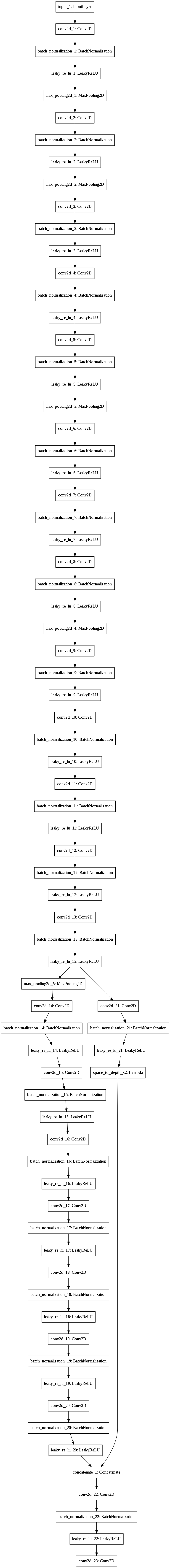Note: On some computers, you may see a warning message from Keras. Don’t worry about it if you do–it is fine.

Reminder: this model converts a preprocessed batch of input images (shape: (m, 608, 608, 3)) into a tensor of shape (m, 19, 19, 5, 85) as explained in Figure (2).

### 3.3 - Convert output of the model to usable bounding box tensors

The output of yolo_model is a (m, 19, 19, 5, 85) tensor that needs to pass through non-trivial processing and conversion. The following cell does that for you.

yolo_outputs = yolo_head(yolo_model.output, anchors, len(class_names))


You added yolo_outputs to your graph. This set of 4 tensors is ready to be used as input by your yolo_eval function.

### 3.4 - Filtering boxes

yolo_outputs gave you all the predicted boxes of yolo_model in the correct format. You’re now ready to perform filtering and select only the best boxes. Lets now call yolo_eval, which you had previously implemented, to do this.

scores, boxes, classes = yolo_eval(yolo_outputs, image_shape)


### 3.5 - Run the graph on an image

Let the fun begin. You have created a (sess) graph that can be summarized as follows:

1. yolo_model.input is given to yolo_model. The model is used to compute the output yolo_model.output
2. yolo_model.output is processed by yolo_head. It gives you yolo_outputs
3. yolo_outputs goes through a filtering function, yolo_eval. It outputs your predictions: scores, boxes, classes

Exercise: Implement predict() which runs the graph to test YOLO on an image.
You will need to run a TensorFlow session, to have it compute scores, boxes, classes.

The code below also uses the following function:

image, image_data = preprocess_image("images/" + image_file, model_image_size = (608, 608))


which outputs:

• image: a python (PIL) representation of your image used for drawing boxes. You won’t need to use it.
• image_data: a numpy-array representing the image. This will be the input to the CNN.

Important note: when a model uses BatchNorm (as is the case in YOLO), you will need to pass an additional placeholder in the feed_dict {K.learning_phase(): 0}.

def predict(sess, image_file):
"""
Runs the graph stored in "sess" to predict boxes for "image_file". Prints and plots the preditions.

Arguments:
sess -- your tensorflow/Keras session containing the YOLO graph
image_file -- name of an image stored in the "images" folder.

Returns:
out_scores -- tensor of shape (None, ), scores of the predicted boxes
out_boxes -- tensor of shape (None, 4), coordinates of the predicted boxes
out_classes -- tensor of shape (None, ), class index of the predicted boxes

Note: "None" actually represents the number of predicted boxes, it varies between 0 and max_boxes.
"""

# Preprocess your image
image, image_data = preprocess_image("/home/hansry/Python/DL/4-3/images/" + image_file, model_image_size = (608, 608))

# Run the session with the correct tensors and choose the correct placeholders in the feed_dict.
# You'll need to use feed_dict={yolo_model.input: ... , K.learning_phase(): 0})
### START CODE HERE ### (≈ 1 line)
out_scores, out_boxes, out_classes=sess.run(
[scores, boxes, classes],
feed_dict={
yolo_model.input: image_data,
K.learning_phase(): 0
})
### END CODE HERE ###

# Print predictions info
print('Found {} boxes for {}'.format(len(out_boxes), image_file))
# Generate colors for drawing bounding boxes.
colors = generate_colors(class_names)
# Draw bounding boxes on the image file
draw_boxes(image, out_scores, out_boxes, out_classes, class_names, colors)
# Save the predicted bounding box on the image
image.save(os.path.join("out", image_file), quality=90)
# Display the results in the notebook
output_image = scipy.misc.imread(os.path.join("out", image_file))
imshow(output_image)

return out_scores, out_boxes, out_classes

for i in range(1,110):
if i<10:
image_file="000"+str(i)+".jpg"
elif i>=10 and i<100:
image_file="00"+str(i)+".jpg"
elif i>=100:
image_file="0"+str(i)+".jpg"
out_scores, out_boxes, out_classes = predict(sess,image_file)

Found 0 boxes for 0001.jpg
Found 0 boxes for 0002.jpg
Found 3 boxes for 0003.jpg
car 0.69 (347, 289) (445, 321)
car 0.70 (230, 307) (317, 354)
car 0.73 (671, 284) (770, 315)
Found 4 boxes for 0004.jpg
car 0.63 (400, 285) (515, 327)
car 0.66 (95, 297) (227, 342)
car 0.68 (1, 321) (121, 410)
car 0.72 (539, 277) (658, 318)
Found 5 boxes for 0005.jpg
car 0.64 (207, 297) (338, 340)
car 0.65 (741, 266) (918, 313)
car 0.67 (15, 313) (128, 362)
car 0.72 (883, 260) (1026, 303)
car 0.75 (517, 282) (689, 336)
Found 2 boxes for 0006.jpg
car 0.72 (470, 286) (686, 343)
car 0.72 (72, 320) (220, 367)
Found 2 boxes for 0007.jpg
car 0.67 (1086, 243) (1225, 312)
car 0.78 (468, 292) (685, 353)
Found 2 boxes for 0008.jpg
truck 0.63 (852, 252) (1083, 330)
car 0.78 (1082, 275) (1275, 340)
Found 0 boxes for 0009.jpg
Found 1 boxes for 0010.jpg
truck 0.66 (736, 266) (1054, 368)
Found 2 boxes for 0011.jpg
truck 0.73 (727, 269) (1054, 376)
car 0.85 (6, 336) (212, 457)
Found 2 boxes for 0012.jpg
car 0.77 (792, 279) (1163, 408)
car 0.87 (539, 330) (998, 459)
Found 1 boxes for 0013.jpg
truck 0.65 (718, 276) (1053, 385)
Found 1 boxes for 0014.jpg
truck 0.64 (715, 274) (1056, 385)
Found 1 boxes for 0015.jpg
truck 0.72 (713, 275) (1086, 386)
Found 1 boxes for 0016.jpg
truck 0.63 (708, 276) (1106, 388)
Found 3 boxes for 0017.jpg
truck 0.64 (666, 274) (1063, 392)
car 0.79 (1103, 300) (1271, 356)
car 0.82 (1, 358) (183, 427)
Found 2 boxes for 0018.jpg
car 0.76 (71, 362) (242, 419)
car 0.77 (340, 339) (553, 401)
Found 2 boxes for 0019.jpg
truck 0.64 (685, 275) (1050, 396)
car 0.85 (16, 377) (450, 559)
Found 1 boxes for 0020.jpg
truck 0.75 (538, 286) (926, 413)
Found 2 boxes for 0021.jpg
car 0.62 (691, 292) (914, 403)
truck 0.72 (88, 317) (493, 450)
Found 2 boxes for 0022.jpg
car 0.65 (894, 302) (980, 348)
car 0.79 (751, 318) (879, 370)
Found 0 boxes for 0023.jpg
Found 0 boxes for 0024.jpg
Found 1 boxes for 0025.jpg
car 0.65 (664, 296) (705, 321)
Found 0 boxes for 0026.jpg
Found 0 boxes for 0027.jpg
Found 1 boxes for 0028.jpg
car 0.72 (711, 303) (792, 368)
Found 1 boxes for 0029.jpg
truck 0.65 (698, 282) (781, 336)
Found 0 boxes for 0030.jpg
Found 1 boxes for 0031.jpg
car 0.67 (187, 316) (313, 409)
Found 0 boxes for 0032.jpg
Found 1 boxes for 0033.jpg
car 0.62 (899, 279) (964, 306)
Found 2 boxes for 0034.jpg
traffic light 0.61 (200, 107) (228, 170)
car 0.70 (179, 326) (312, 424)
Found 1 boxes for 0035.jpg
car 0.62 (1084, 278) (1194, 319)
Found 2 boxes for 0036.jpg
car 0.65 (211, 313) (349, 402)
car 0.73 (1014, 274) (1201, 338)
Found 1 boxes for 0037.jpg
car 0.63 (326, 302) (419, 365)
Found 0 boxes for 0038.jpg
Found 1 boxes for 0039.jpg
car 0.67 (312, 301) (398, 364)
Found 1 boxes for 0040.jpg
car 0.61 (330, 299) (415, 363)
Found 1 boxes for 0041.jpg
car 0.65 (341, 294) (415, 367)
Found 0 boxes for 0042.jpg
Found 1 boxes for 0043.jpg
car 0.76 (118, 312) (237, 384)
Found 1 boxes for 0044.jpg
car 0.61 (551, 283) (624, 329)
Found 2 boxes for 0045.jpg
traffic light 0.70 (383, 40) (416, 101)
traffic light 0.73 (569, 33) (604, 102)
Found 0 boxes for 0046.jpg
Found 0 boxes for 0047.jpg
Found 0 boxes for 0048.jpg
Found 0 boxes for 0049.jpg
Found 0 boxes for 0050.jpg
Found 2 boxes for 0051.jpg
car 0.68 (151, 323) (247, 379)
traffic light 0.72 (500, 79) (532, 138)
Found 0 boxes for 0052.jpg
Found 0 boxes for 0053.jpg
Found 2 boxes for 0054.jpg
car 0.63 (726, 293) (800, 353)
car 0.72 (786, 292) (941, 410)
Found 1 boxes for 0055.jpg
car 0.73 (758, 277) (904, 389)
Found 0 boxes for 0056.jpg
Found 0 boxes for 0057.jpg
Found 0 boxes for 0058.jpg
Found 3 boxes for 0059.jpg
car 0.77 (0, 307) (257, 464)
car 0.82 (570, 277) (864, 417)
car 0.86 (86, 319) (527, 493)
Found 0 boxes for 0060.jpg
Found 0 boxes for 0061.jpg
Found 0 boxes for 0062.jpg
Found 0 boxes for 0063.jpg
Found 0 boxes for 0064.jpg
Found 1 boxes for 0065.jpg
car 0.69 (380, 270) (462, 324)
Found 2 boxes for 0066.jpg
traffic light 0.62 (532, 68) (564, 113)
car 0.77 (372, 281) (454, 333)
Found 2 boxes for 0067.jpg
traffic light 0.65 (535, 60) (570, 105)
car 0.70 (369, 280) (454, 345)
Found 3 boxes for 0068.jpg
traffic light 0.64 (378, 87) (405, 146)
traffic light 0.64 (536, 60) (572, 108)
car 0.66 (367, 288) (450, 348)
Found 3 boxes for 0069.jpg
traffic light 0.60 (537, 62) (577, 109)
car 0.62 (367, 289) (450, 346)
traffic light 0.63 (379, 87) (407, 147)
Found 1 boxes for 0070.jpg
car 0.65 (369, 291) (452, 354)
Found 1 boxes for 0071.jpg
truck 0.70 (87, 287) (569, 450)
Found 6 boxes for 0072.jpg
traffic light 0.61 (535, 65) (572, 111)
traffic light 0.62 (378, 91) (406, 148)
car 0.62 (291, 301) (357, 351)
truck 0.64 (1049, 263) (1280, 399)
car 0.64 (0, 331) (84, 449)
car 0.66 (368, 292) (450, 357)
Found 2 boxes for 0073.jpg
car 0.74 (145, 313) (248, 374)
car 0.85 (503, 299) (858, 421)
Found 3 boxes for 0074.jpg
traffic light 0.60 (380, 91) (407, 147)
car 0.61 (365, 294) (450, 346)
car 0.87 (31, 319) (424, 485)
Found 1 boxes for 0075.jpg
car 0.75 (151, 315) (246, 372)
Found 4 boxes for 0076.jpg
traffic light 0.62 (381, 93) (407, 146)
car 0.66 (246, 298) (336, 366)
car 0.70 (369, 292) (451, 357)
car 0.75 (150, 313) (245, 375)
Found 5 boxes for 0077.jpg
traffic light 0.60 (536, 65) (571, 112)
traffic light 0.60 (380, 92) (407, 147)
car 0.70 (243, 296) (345, 368)
car 0.71 (368, 292) (450, 356)
car 0.75 (150, 313) (245, 374)
Found 4 boxes for 0078.jpg
traffic light 0.62 (380, 92) (407, 146)
car 0.65 (367, 293) (453, 353)
car 0.70 (242, 295) (339, 367)
car 0.75 (151, 313) (245, 373)
Found 4 boxes for 0079.jpg
traffic light 0.61 (535, 65) (571, 111)
traffic light 0.61 (378, 92) (406, 148)
car 0.72 (235, 298) (327, 367)
car 0.76 (151, 314) (243, 373)
Found 4 boxes for 0080.jpg
traffic light 0.61 (379, 92) (407, 147)
car 0.64 (5, 309) (188, 416)
car 0.71 (237, 298) (324, 366)
car 0.79 (714, 282) (916, 362)
Found 3 boxes for 0081.jpg
car 0.68 (187, 309) (301, 381)
car 0.76 (612, 293) (722, 353)
car 0.79 (25, 328) (141, 398)
Found 3 boxes for 0082.jpg
traffic light 0.61 (380, 92) (408, 147)
car 0.62 (585, 287) (660, 335)
car 0.70 (410, 282) (609, 388)
Found 3 boxes for 0083.jpg
traffic light 0.63 (380, 91) (407, 148)
car 0.64 (0, 328) (82, 447)
car 0.72 (609, 280) (888, 397)
Found 2 boxes for 0084.jpg
traffic light 0.62 (378, 91) (406, 150)
car 0.70 (990, 272) (1270, 381)
Found 2 boxes for 0085.jpg
traffic light 0.61 (535, 64) (571, 114)
traffic light 0.61 (378, 91) (406, 150)
Found 2 boxes for 0086.jpg
traffic light 0.60 (378, 92) (407, 150)
traffic light 0.61 (536, 65) (572, 113)
Found 3 boxes for 0087.jpg
truck 0.60 (0, 315) (93, 404)
traffic light 0.60 (536, 66) (572, 112)
traffic light 0.61 (382, 92) (410, 149)
Found 1 boxes for 0088.jpg
traffic light 0.61 (377, 92) (406, 150)
Found 0 boxes for 0089.jpg
Found 0 boxes for 0090.jpg
Found 1 boxes for 0091.jpg
traffic light 0.71 (300, 76) (333, 159)
Found 1 boxes for 0092.jpg
traffic light 0.62 (232, 25) (266, 95)
Found 1 boxes for 0093.jpg
car 0.60 (361, 313) (414, 341)
Found 0 boxes for 0094.jpg
Found 0 boxes for 0095.jpg
Found 1 boxes for 0096.jpg
car 0.68 (202, 319) (301, 369)
Found 2 boxes for 0097.jpg
car 0.69 (74, 330) (188, 395)
car 0.69 (235, 315) (336, 375)
Found 3 boxes for 0098.jpg
car 0.60 (747, 289) (811, 356)
car 0.63 (836, 291) (968, 405)
car 0.81 (898, 315) (1113, 452)
Found 3 boxes for 0099.jpg
car 0.75 (1046, 352) (1279, 608)
car 0.78 (859, 316) (972, 427)
car 0.86 (921, 336) (1120, 476)
Found 0 boxes for 0100.jpg
Found 0 boxes for 0101.jpg
Found 1 boxes for 0102.jpg
bus 0.79 (180, 259) (304, 362)
Found 1 boxes for 0103.jpg
bus 0.74 (0, 286) (203, 420)
Found 2 boxes for 0104.jpg
traffic light 0.62 (241, 24) (273, 97)
truck 0.82 (0, 223) (291, 421)
Found 0 boxes for 0105.jpg
Found 1 boxes for 0106.jpg
car 0.62 (1200, 287) (1276, 432)
Found 1 boxes for 0107.jpg
car 0.60 (376, 309) (421, 335)
Found 0 boxes for 0108.jpg
Found 0 boxes for 0109.jpg



import cv2
from cv2 import VideoWriter,VideoWriter_fourcc,imread,resize
img_root="out/"
fps=15
fourcc=VideoWriter_fourcc(*"MJPG")
videoWriter=cv2.VideoWriter('Video/car.avi',fourcc,fps,(1280,720))

for i in range(1,110):
if i<10:
image_file="000"+str(i)+".jpg"
elif i>=10 and i<100:
image_file="00"+str(i)+".jpg"
elif i>=100:
image_file="0"+str(i)+".jpg"
frame=cv2.imread(img_root+image_file)
print (image_file)

videoWriter.write(frame)

videoWriter.release()